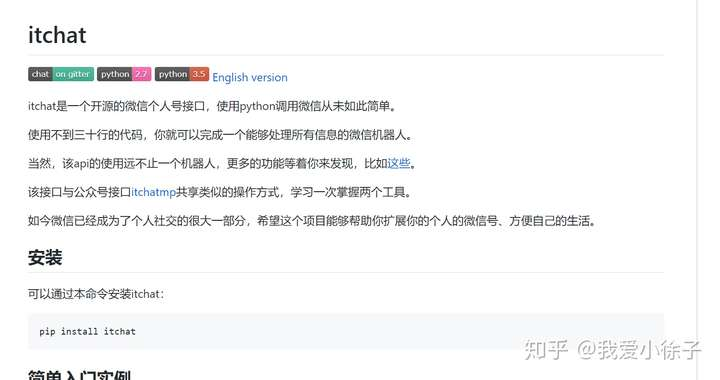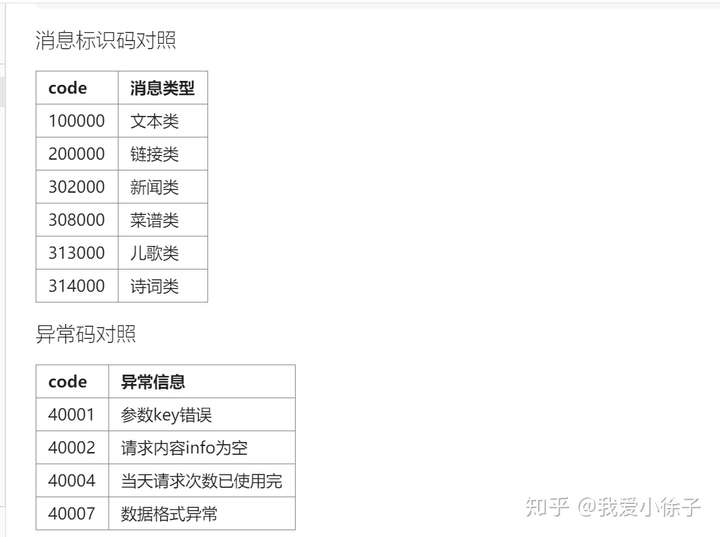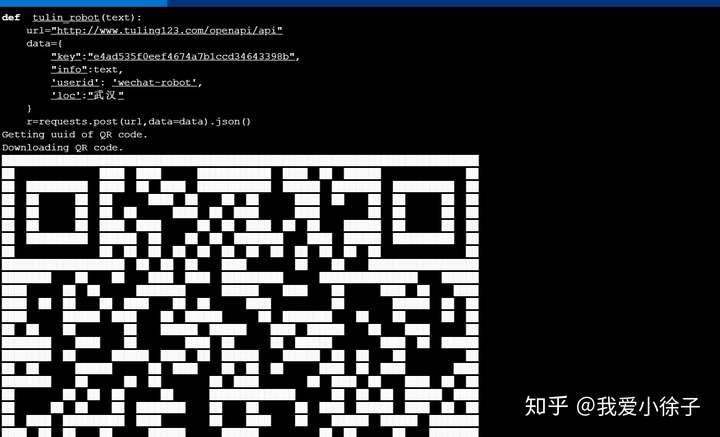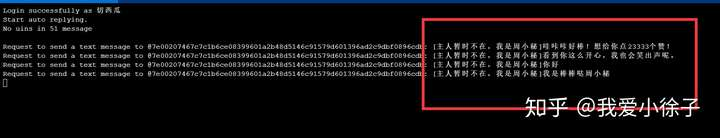python:用itchat打造属于自己的微信聊天机器人，挂在自己的服务器上24小时运行，很简单~

0 人赞了该文章这是成功的示意图：pip  install   itchat

import itchat

#!/usr/bin/python
#coding=utf8
import itchat
import  time
# 自动回复
# 封装好的装饰器，当接收到的消息是Text，即文字消息
@itchat.msg_register('Text')
# 当消息不是由自己发出的时候
return  u"[主人暂时不在，我是周小秘]{}".format(msg['Text'])
# 回复给好友
if __name__ == '__main__':
itchat.run(debug=True)import  requests
import  json
def  tulin_robot(text):
url="http://www.tuling123.com/openapi/api"
data={
"key":"*****************",#自己机器人apikey值
"info":text,#从微信传输过来的文本内容
'userid': 'wechat-robot',
'loc':"武汉"
}
r=requests.post(url,data=data).json()
code=r["code"]
"""100000  文本类
200000  链接类
302000  新闻类
308000  菜谱类
313000  儿歌类
314000  诗词类"""
if  code==  302000:
return  r["text"],r["list"]
if code==  100000:
return  r["text"]
if   code==200000:
return r["text"],r["url"]
if   code==313000:
return r["text"],r["function"]
if   code==314000:
return  r["text"],r["function"]
if   code==308000:
return r["text"],r["list"]
print(tulin_robot("武汉天气"))#调用函数tulin_robot,并输出返回内容

#!/usr/bin/python
#coding=utf8
import itchat
import  time
import   requests
import   json
def  tulin_robot(text):
url="http://www.tuling123.com/openapi/api"
data={
"info":text,
'userid': 'wechat-robot',
'loc':"武汉"
}
#!/usr/bin/python
#coding=utf8
import itchat
import  time
import   requests
import   json
def  tulin_robot(text):
url="http://www.tuling123.com/openapi/api"
data={
"info":text,
'userid': 'wechat-robot',
'loc':"武汉"
}
r=requests.post(url,data=data).json()
code=r["code"]
"""100000   文本类
200000   链接类
302000   新闻类
308000   菜谱类
313000   儿歌类
314000   诗词类"""
if  code==  302000:
return  r["text"],r["list"]
if code==  100000:
return  r["text"]
if   code==200000:
return r["text"],r["url"]
if   code==313000:
return r["text"],r["function"]
if   code==314000:
return  r["text"],r["function"]
if   code==308000:
return r["text"],r["list"]
# 自动回复
# 封装好的装饰器，当接收到的消息是Text，即文字消息
@itchat.msg_register('Text')
# 当消息不是由自己发出的时候
return  u"[主人暂时不在，我是周小秘]{}".format(tulin_robot(msg['Text']))
# 回复给好友
if __name__ == '__main__':

itchat.run(debug=True)

python3    auto_wechat.py

screen python3 auto_wechat.py \$ 即可保持程序一直在服务器后台运行。screen  ls

python和linux的功能十分强大，还需要我们继续学习。有任何问题可以评论，我看见就会回复，也可以私信我。